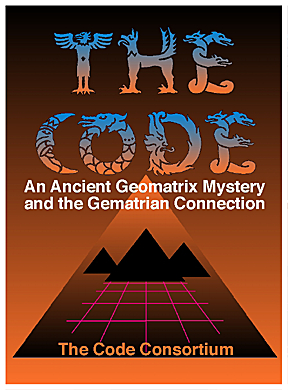"THE CODE" OF CARL MUNCK
AND ANCIENT GEMATRIAN NUMBERS
PART FIVE

The King's Chamber and the Sarcophagus
of the Great Pyramid

by Michael Lawrence Morton

with Contributions from James Furia and Joseph E. Mason

Overview

by Joseph E. Mason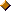Recently, Michael Lawrence Morton composed an article showing a possible "Code" connection to the dimensions of the King's Chamber and the Sarcophagus inside the Great Pyramid.  James Furia read Michael's article, and realized that there were even more connections.  This article starts with Michael's article. James Furia's additional findings and a few other comments follow.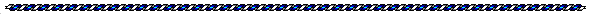The King's Chamber and the Sarcophagus
of the Great Pyramid

by Michael Lawrence Morton

Length and Width of The King's ChamberOn page 54, in the book "The Great Pyramid Decoded", by E. Raymond Capt, the length of The King's Chamber is given in "pyramid inches" as 412.13186, the width as 206.06593, and the height as 230.38871. We are told that a "pyramid inch" is slightly larger than a regular inch, a ratio of approximately 1.000965 or so.

As soon as I saw the first 3 digits of the given length (above) . . . 412 . . . I thought of the decimal harmonic of the Surface Area On A Sphere . . . 4125296125 (to ten digits). This figure comes from a geometry 'formula'; using the RADIAN (deg) *as the radius* of a 'generic sphere'. In other words, we are taught (hopefully) in school that the "formula for the Area Of A Circle"is . . . "Pi x 'r' Squared."

What I'm saying here is to actually substitute the figure 57.29577951 (degrees of arc) for the "r" in that generic formula we learn in school. The RADIAN (deg) is equal to (assuming 360 degrees on one complete circumference) 57.29577951 degrees-of-arc on any true circumference. This curved length . . . the RADIAN (deg) . . . is equal, as close as possible, to the straight-line length of the actual radius of that *same* circle or sphere.

The formula (generic) for Surface Area On A Sphere is :

4 x Pi x ('r' Squared)

So ; if we substitute 57.29577951 (deg) for "r" . . .

4 x 3.141592654 x (57.29577951 x 57.29577951)

= 41252.96125 . . . an actual number value we can use.

What would the ratio be, of "pyramid inch" to regular inch, if we look at the possibility of the length of The King's Chamber as 412.5296125 regular inches ?

412.5296125 / 412.13186 = 1.00096511

Yes; this is very close to the estimate of 1.000965 given above.

I think we have probably found something very significant here. The work of Carl P. Munck (self-published) has proven the reality of a lost, deeply ancient . . . and evidently suppressed . . . advanced planetary mathematical matrix involved in precise latitude and longitude placements of pyramids, mounds, stone circles, monuments, and earthen effigies. This advanced mathematical matrix has also been found to be frequently *encoded* into the structural designs and into the internal geometry and measurements of the structures themselves.

As noted above, the width of The King's Chamber is exactly half its length. So, the width in terms of regular inches would be a decimal harmonic of the Surface Area On A Hemisphere . . . 2062648063 (to ten digits).

Area of The King's Chamber FloorTaking the length times the width, in regular inches, will give us the area in regular square inches, of the floor :

412.5296125 x 206.2648063 = 85090.34062 Sq. in.

If we now divide this area by the Square of the RADIAN (deg) :

85090.34062 / 3282.80635 = 25.92

We find here a decimal harmonic of one complete Precession Cycle of the Equinoxes of Earth in terms of years. This would be :

25920 / 1000 = 25.92 (years)

Already, we can see an apparent encoding of important information, in the actual dimensions of The King's Chamber, in terms of regular inches. This information includes geometry, math constants, base ten, and Earth's Precession Cycle.

The Height of the King's ChamberWhat about the height of this room? Above, we are given the figure of 230.38871 "pyramid inches" as the height. I'm going to say the height is 230.6110641 regular inches, because I think the Square Root of 5 was used here due to its association with the speed-of-light in air (Earth's atmosphere) . . . and this is verified by our modern-day measurement of 186234.0949 miles per second (see Part One of this series of articles). Carl P. Munck's work has shown that certain 'tangents' . . . the trigonometry function . . . were extremely important to the very ancient-but-advanced matrix discussed earlier.

Two of these very important tangents were encoded in the "gematrian numbers" used in the matrix: plus/minus 3.077683537 and . . . plus/minus 0.726542528. Notice that when these two tangents are multiplied, we get the Square Root of 5 :

0.726542528 x 3.077683537 = 2.236067978

= Square Root of 5

Now, the tangent of the speed-of-light in air . . . 186234.0949 miles per second . . . is -2.236061962, practically a match to the Square Root of 5.

Returning to The King's Chamber, here is how I came up with the figure of 230.6110641 regular inches for the height:

103.1324031 x 2.236067978 = 230.6110641

What is 103.1324031?  It is a decimal harmonic of the Area Of A Circle:

Pi x ( 'R' x 'R') = 10313.24031

Pi x (57.29577951 x 57.29577951) = 10313.24031

Volume of the King's ChamberTo find the volume of The King's Chamber in terms of regular cubic inches, we multiply length x width x height:

412.5296125 x 206.2648063 x 230.6110641 = 19622774

At this point, I'm not sure what that particular figure means, or whether or not it is 'significant'. As an alpha numerologist, however, I can see an interesting 'mosaic' presented if we separate the 8 digits into 196, 227, and 74.

196 = Square of 14

227 = Pi (classic approximation of 22 / 7)

74 = sum of the word CROSS, and sum of the name JESUS

(I use all 26 letter positions, without 'reducing' to single digits)

If we look at the number 7 as a "diameter", there is a correlation among these 3 'number entities' that I find striking. A "square of 14" can be visualized as two "diameters of 7" at right angles to one another . . . in a cross. The "22" of the (22 / 7) Pi approximation is the circumference of a circle around the cross, and the "7" from that approximation can be seen as a "third axis" intersecting the 2-D cross, again at right angles, providing a model of 3-D space.

Adding the 3 'number entities' :

196 + 227 + 74 = 497

666 - 497 = 169, the Square of 13 . . . referring to the 13 "tones" of a complete chromatic scale in music, ending with the first note of the next octave. This is also the 13 "tones of creation" from the Mayan tradition.

The number 153 is very important (see Part One), and I won't go into all of the aspects here, except to point out that it is half of 306, which in turn is the sum of 144 + 162 . . . and these numbers are decimal harmonics of Bruce Cathie's speed-of-light equivalents of 144000 nautical miles per 'grid second' and 162000 nautical miles per second.

We also find 306 as azimuth node number 17 on the 'wheel of 20 nodes', where the nodes are 18 degrees apart. The 20-part wheel (see work of Joe Mason in Part One) is also a symbol of the Mayan Haab Calendar cycle of 18 'months' of 20 days each month.

17 x 9 = 153

Now . . . 497 - 153 = 344, which is always July 4th on the Mayan Haab Calendar, because Day Number 360 is always July 20th. (I will note here that the 153rd course of masonry from the ground, on The Great Pyramid, is 360 FEET above the ground . . . see work of Jimi Furia).

344 = 227 + 117, and 117 can be visualized as "half-Pi", or (11 / 7) approximation.

Also, 117 = 19.5 x 6, where there are 6 places at 19.5 degrees north and south, at which 6 vertices of two interlocking tetrahedra touch the surface of a circumscribing sphere ... assuming the remaining two vertices are touching at the poles. This is a model of "hyperdimensional physics", as discussed by researcher and journalist Richard C. Hoagland, as applied to certain dynamics of rotating celestial bodies.

The SarcophagusAgain, in the book "The Great Pyramid Decoded", by E. Raymond Capt, on page 54 we see dimensions given in "pyramid inches" for The Coffer (or Sarcophagus) that sits at the west end of The King's Chamber :

length 89.80568, width 38.69843, and height 41.21319

I think these dimensions, in terms of regular inches, are:

length 90, width 38.78509448, and height 41.25296125 . . . and here's why:

90 x 38.78509448 x 41.25296125 = 144,000

We see that these dimensions in regular inches, would multiply to exactly 144,000 . . . a major 'gematrian' number referring to "light", and referring to *speed-of-light* (theoretical maximum) according to the work of Bruce Cathie. See his book, "The Harmonic Conquest of Space." Of course, there is the Biblical reference to 144,000 as well.

So; we've now established evidence of the encoding of information relating to light, or to the 'speed-of-light', in the actual dimensions of The King's Chamber and in the actual dimensions of The Sarcophagus, in terms of regular inches.

SummaryTo summarize; looking at the dimensions of the The King's Chamber and at the dimensions of The Sarcophagus within it, in terms of regular inches, reveals evidence of the encoding of advanced information, including circle/sphere geometry, the use of 360 degrees-of-arc on one complete circumference, the math constants of Pi and the RADIAN (deg), the use of 'base ten', the Precession Cycle of Earth in years, the speed-of-light in Earth's atmosphere in terms of miles per second, and the Square Root of 5 as the TANGENT of said light speed.

Michael Lawrence Morton

July 28, 1998

 Michael Lawrence Morton graduated from Franklin and Marshall College (Lancaster, Pennsylvania) in 1973 with a B.A. degree in English Literature. He is an independent researcher into lost and suppressed human history and culture. He is an archeocryptographer and an alpha-numerologist.

To contact Michael Lawrence Morton, please e-mail him at Milamo@aol.com,

or phone him at 412-921-9116 in Pittsburgh, PA.Note

This article by Michael Lawrence Morton contains some of his original work. Although he wants to share the material, his wish is that his copyright of the material be respected.  The article can be shared, but please include the copyright notice and this statement with it.I was told that the inside dimensions of the kings chamber, were/are 17 feet by 34 feet. Of course this is a rounded estimation, but close to the amount of inches you are displaying. We know that 17 is related to the Great Pyramid . . . the entrance to it is at the 17th course not the 19th. I blew up the picture of the front and counted 17 courses to the entrance.

WOW! . . . LOOK -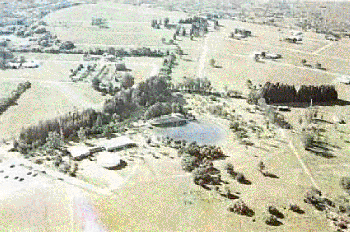One of the numbers I remember from Warm Mineral Springs is 34.377468.

34.377468 feet IS 412.52596 inches!!!!

34.377468 is also 108/pi !!!

WOW!  I think we finally have the inner measurements.Carl Munck's numbers for Warm Mineral Springs is in Part Four of this series of articles. For convenience, that part of the article is reproduced below:

_____________________________________

Carl Munck's Numbers for Warm Mineral Springs

3/5 Radian (34.37746771)  is revealed  again at Tikal, in the West Giza longitude of Temple III (120 degrees 45 minutes 34.37746 seconds)

AND

(2) is the Matrix C.I. (Coordinate Intersect) of Warm Mineral Springs in Florida, now suspected as the fabled Fountain of Youth,

AND

(3) The C.I. of Florida's Mound Key complex.

[and then, more:]

Warm Mineral Springs in the Global Matrix System -

113 degrees 23 minutes 37.59" WEST GIZA - (97716.09785)

C.I.  = 34.37746771

(WMSLA-2842.446063)

27 degrees 03 minutes 35.09" N.

Notice that the matrix C.I. of Warm Mineral Springs is a mathematical constant in that its value exactly equals 3/5ths of 57.29577951 radian degree.

O.K. Now, lets apply our gematrian numbers to Warm Mineral Springs:

WMSX (34.37746771) X PI = 108

A direct link with the gematrian realm!

Then, simply apply our basic numbers to the puzzle. You know, 1, 2, 3, 4, 5, and so on . . .Only make them pi-multiples . . . Pi,  2 pi,  3 pi,  4 pi, 5 pi, 6 pi, etc. Now we are treated to:

WMSX  x Pi = 108

x 2pi = 216

x 3pi = 324

x 4pi = 432

x 5pi = 540 (Tangent - 0 )

x 6pi = 648...

MORE gematrian numbers, stacked up like cordwood. What do they show us?

_____________________________________Yes !!!! The length of The King's Chamber is exactly 34.37746771 FEET !!!!

3/5ths of the RADIAN (deg) . . . Warm Mineral Springs C.I. !!!!!!

And the height of The Sarcophagus is exactly 3.437746771 FEET !!!!!!

Base ten in action !

WOW ! IS RIGHT !! HOLY SMOKES !!!!!!

Volume of The King's ChamberI think I've found the Height of The King's Chamber, in terms of regular inches :

230.7334064 inches

This is approximately 0.122 inches more than my previous figure.

I used Carl P. Munck's area for the Sarsen Circle at Stonehenge, in terms of Square FEET . . . 7441.506403 . . .

7441.506403 / 1000 x (Pi Cubed) = 230.7334064

This enables the following calculation for Volume of The King's Chamber :

230.7334064 x 412.5296125 x 206.2648063 = 19633184.14

(H) (L) (W) Cubic Inches

The number 19633184.14 can be factored exactly into the product of a 'gematrian-Pi' multiple, a Precessional harmonic (base-10), and the Cube of Pi :

(7776 x Pi) x 25.92 x (Pi Cubed) = 19633184.14

ALSO . . .

As Jimi Furia pointed-out earlier today, the Length of The King's Chamber, 412.5296125 regular inches, is exactly equal to :

34.37746771 FEET . . . which is an exact match for the Grid Point Value of the site at Warm Mineral Springs, Florida.

Michael Lawrence MortonThe Golden Coffin

by James FuriaNine Planets, nine Pyramids total at the Giza Complex . . . 9, the root of all Gematria.

The golden mean ratio, 1.618033 (Phi), found in nature and in the Pentagon.

9 / Phi / Phi = 3.4377

= The exact height of the so-called coffin.

Perhaps the coffin is really a resonate mass forged by the harmonic waves of the interior of the chamber.

And as more equations are revealed, we might suspect TIME as recorder of such Geometric anomalies. The surface area of the King's chamber is 590.90515 feet.

590.90515 / Phi = 365.20

= the solar year at a point in time somewhere in between the construction of these monuments and the present time. Carl has been calculating such time counts for years.

James Furia

July 31, 1998The 'Christ Angle'

by Michael Lawrence Morton

This is a brief update on my work with the book, "The Great Pyramid Decoded", by E. Raymond Capt.

E. Raymond Capt gives the "Christ Angle", on page 81, as :

26 deg 18 min 9.7 sec

Look at what happens when we apply Carl P. Munck's system of multiplying (no. of deg) X (no. of min) X (no. of sec) . . .

26 deg x 18 min x 9.666438934 sec = 4523.893421

= 1440 x Pi

In his book, "The Great Pyramid Decoded", Capt says that to form this angle, a line is drawn from The Great Pyramid to (and through) Bethlehem. The angle is measured counter-clockwise, from a line drawn due East from The Great Pyramid. Once again, we see the number 1440 combined with the Pi constant.

The volume of the outer dimensions of The Sarcophagus in The King's Chamber is precisely 144,000 Cubic Inches . . . regular inches, not "pyramid inches" . . . as we have shown very recently. Repeatedly, we are shown the 'gematrian' number 144 and various decimal harmonics of 144, throughout this very ancient (now re-discovered . . . see Munck) planetary matrix.

The Grid Latitude of 'The Face' @ Cydonia, on Mars, is also equal to (1440 x Pi) :

41 deg x 11 min x 10.03080581 sec = 4523.893421 North

= 1440 x Pi

I have calculated a Grid Point Value at Bethlehem of exactly one-tenth the Grid Point Value of 'The Face' @ Cydonia :

656.56127 / 10 = 65.656127

This Bethlehem grid point is based not only on Munck's system, but also on a separate grid system (involving Earth's magnetic field) developed by Bruce Cathie. I actually found a merging-point here of the 'grid systems' of these two researchers.

We have shown, again very recently, that the volume of The King's Chamber . . . in regular inches, not "pyramid inches" . . . is 19633184.14 cubic inches. Notice the following :

19633184.14 / Pi Cubed / Pi Cubed / 311.04 = 65.656127

Again, we have a major 'gematrian' number factoring-in here . . . a decimal harmonic of 31104. Look at what happens when we divide the number 144 into 31104 . . .

31104 / 144 = 216

= 6 Cubed, or 6 x 6 x 6

The number 216 refers us, in turn, to the Grid Latitude of Stonehenge (see Part One), which is the figure 21,600 . . . encoding the polar circumference of Earth in nautical miles AND in minutes of latitude arc :

Latitude of Stonehenge :

51 deg x 10 min x 42.35294118 sec = 21600 North

Michael Lawrence Morton

August 1, 1998The Purpose of the King's Chamber

By James Furia

What is the purpose of the Kings Chamber?

On a quantum level ,what is the difference between a tree, a human, a rock, water, a plant? Matter is determined by the rate of it's vibrations. Because of the laws of adhesion, cohesion, attraction and repulsion, which are all effects of one energy, what differs in a rock or plant or anything, is the rate of its vibrations. So . . . why did the ancients create the King's chamber with dimensions 5 octaves below the speed of sound? Why is it acoustically SO precise?

It is now my belief that the chamber is indeed a particle accelerator! But why? For what reason? Shape-shifting malleable matter from human to any animal, or, in reverse, any alien species, could shape-shift into human form with such a particle accelerator.

This is the reason for the countless depictions of human bodies with animal heads.

Nowhere in Egypt is there a drawing of the human holding his/her animal mask . . .  they are all attached. This is also why they name the stars with animals -  the Zodiacal constellations.

James Furia
January 24, 1999End of Part Five

If you have any questions or comments e-mail JOE MASONReferences

"Measurements throughout the pyramid show that its constructors knew of the
proportions of pi (3.14 . . ), phi or the Golden Mean (1.618), and the "Pythagorean"
triangles thousands of years before Pythagoras, the so-called father of geometry, lived."
Martin Gray

"The casing stones, 144,000 in all, were so brilliant that they could literally be seen
from the mountains of Israel hundreds of miles away."

"The circle formed in that first antechamber, defined by the floor
and the two hanging walls measures 365.25 inches."PART ONE
Introduction

PART TWO
Articles by Michael Lawrence Morton
Article One:  The Face' at Cydonia on Mars
Article Two:  Rennes Le Chateau and Bethlehem
Article Three:  "Faces" On Earth And Mars
Article Four:
Major Discovery - Phi, 18 And Poussin's Secret

PART THREE
The Panther Mound and Sphinx Connection
By Michael Lawrence Morton
First Article:  Carl P. Munck and Zecharia Sitchin
RE : The Great Sphinx
Second Article:  Another Sphinx Mystery (and Panther Mound)

PART FOUR
Dream of the Gematrian Wheel and Carl Munck's Response
By Joseph E. Mason

PART SIX
Three Articles:
The Sphinx, Two Fountains Of Youth,
And The King's Chamber

By James Furia
Looking At Coral Castle
By Michael Lawrence Morton
It is Painfully Obvious
There is a Unified Theory
in the Mathematical Grid of the Ancient Ones

by James Furia

PART SEVEN
The Crop Circle Connection
by Joseph E. Mason

PART EIGHT
The Great Sphinx Knows Earth's 'Polar Dimensions'
by Michael Lawrence Morton

PART NINE
Some Pittsburgh Area 'Code Sites'
by Michael Lawrence Morton

PART TEN
Gettysburg, Pennsylvania "Code" Connections
by Michael Lawrence Morton

PART ELEVEN
Miami: The home Of The Mayans?
by Dee Finney
With Decoding by Michael Lawrence Morton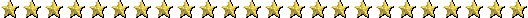Thanks to Ender Design, Inc. for the free graphics from Realm Graphics